# RD Sharma Solutions for Class 8 Chapter 9 Linear Equation in One Variable Exercise 9.4

In Exercise 9.4 of Chapter 9, we shall discuss the concept of applications of linear equations to practical problems (i.e., word problems). RD Sharma Solutions for Class 8 Maths are provided here with all the solutions to the questions given in the textbook in simple language for better understanding of the concept. Our experts at BYJU’S have formulated RD Sharma Solutions to help students boost their confidence level to achieve high marks in their exams. Links are provided below where students can download the pdf easily.

## Download the pdf of RD Sharma for Class 8 Maths Exercise 9.4 Chapter 9 Linear Equation in One Variable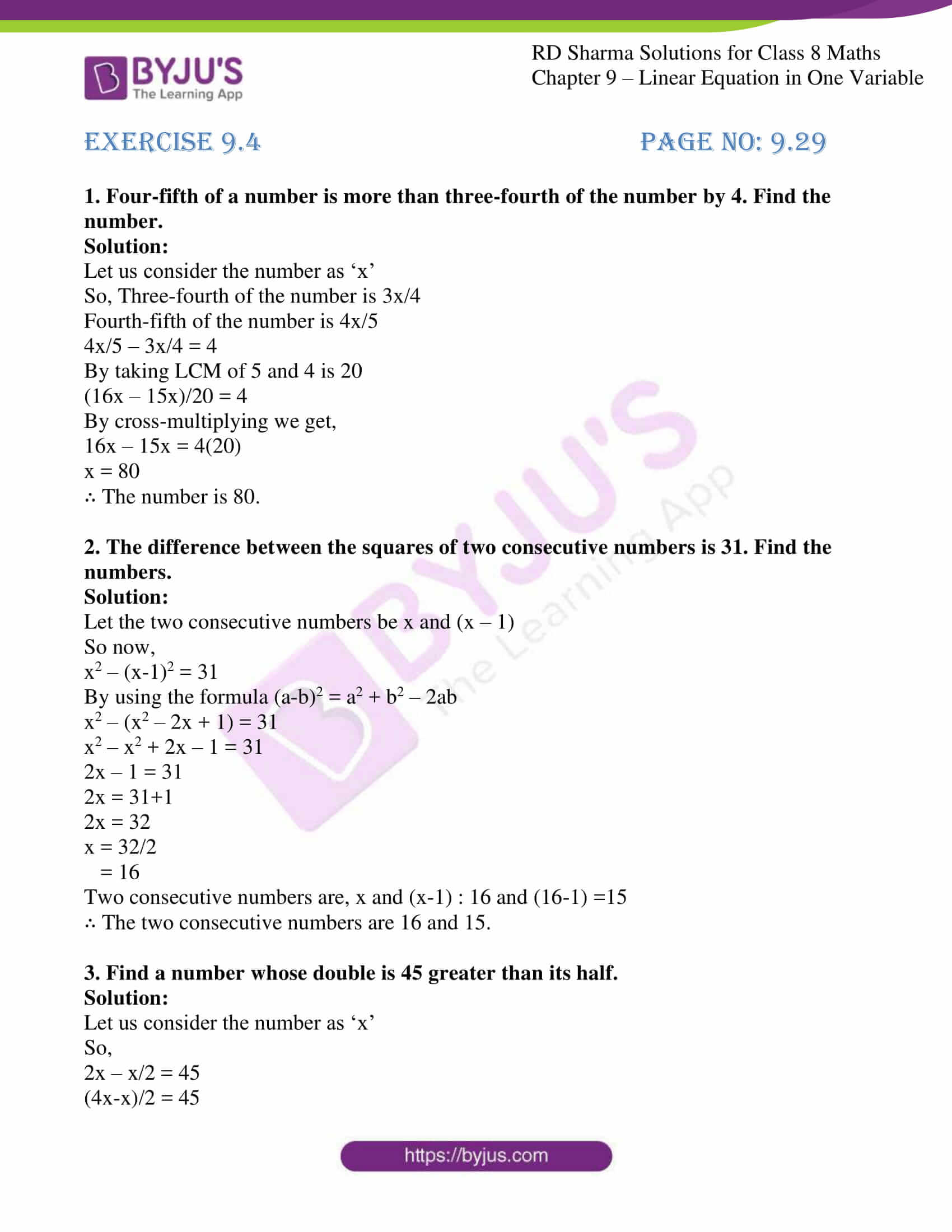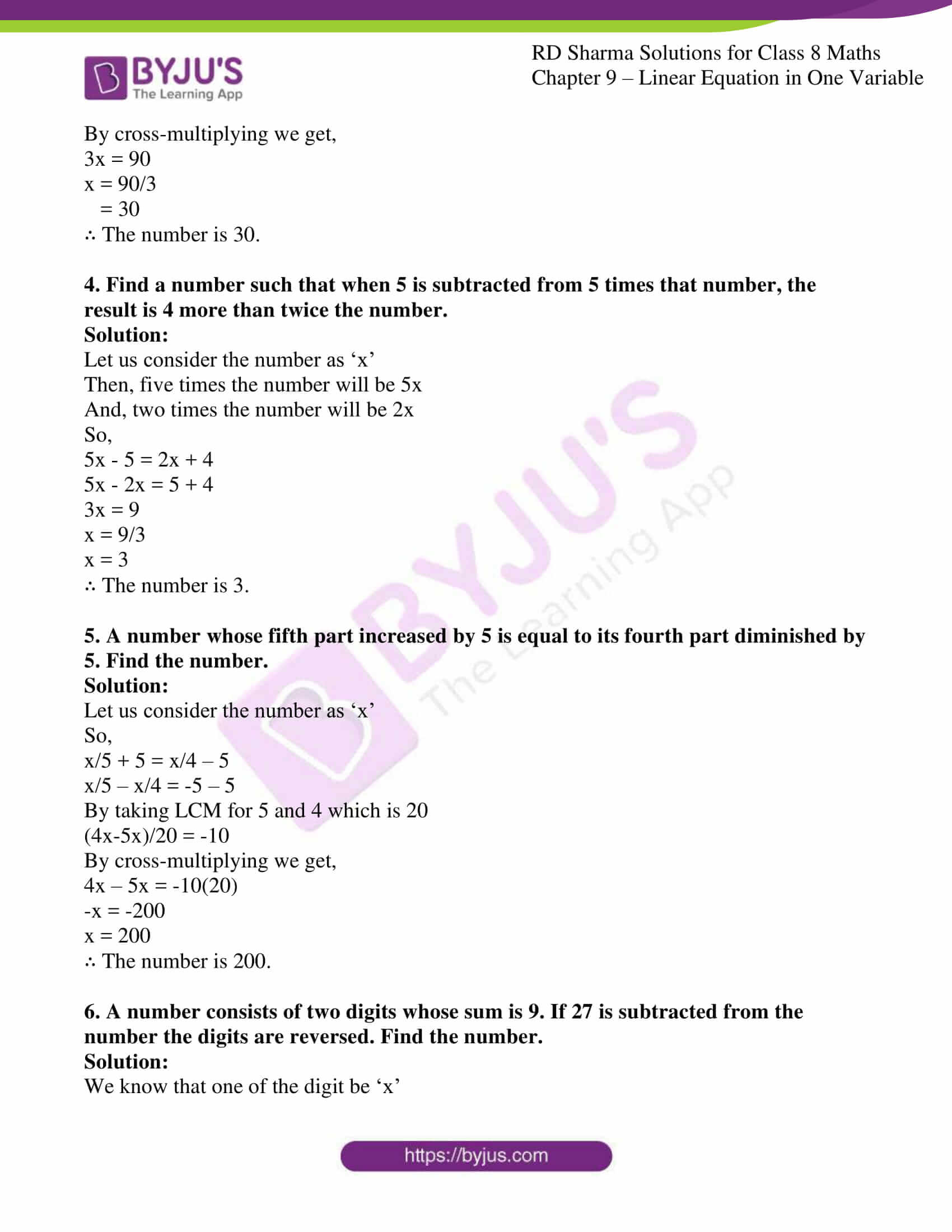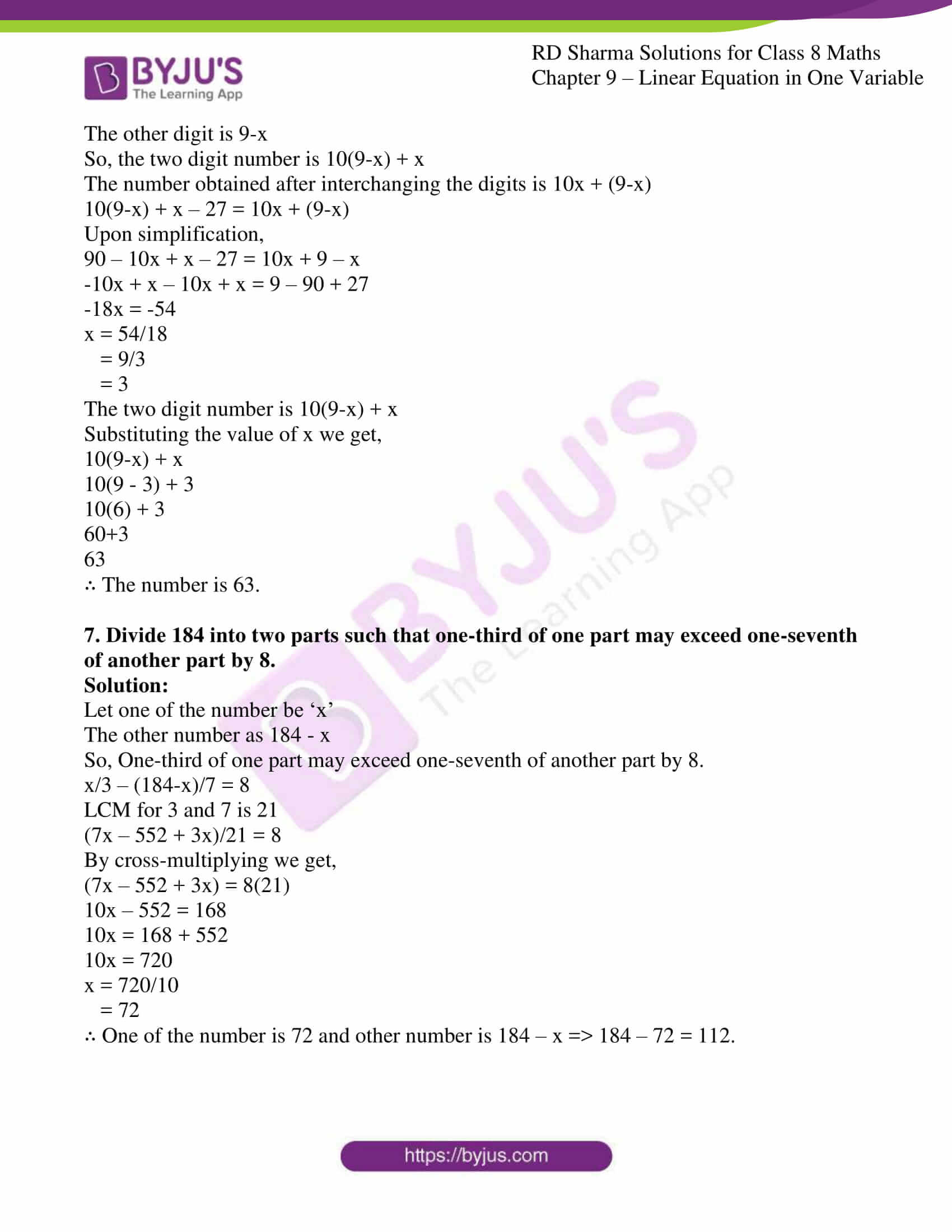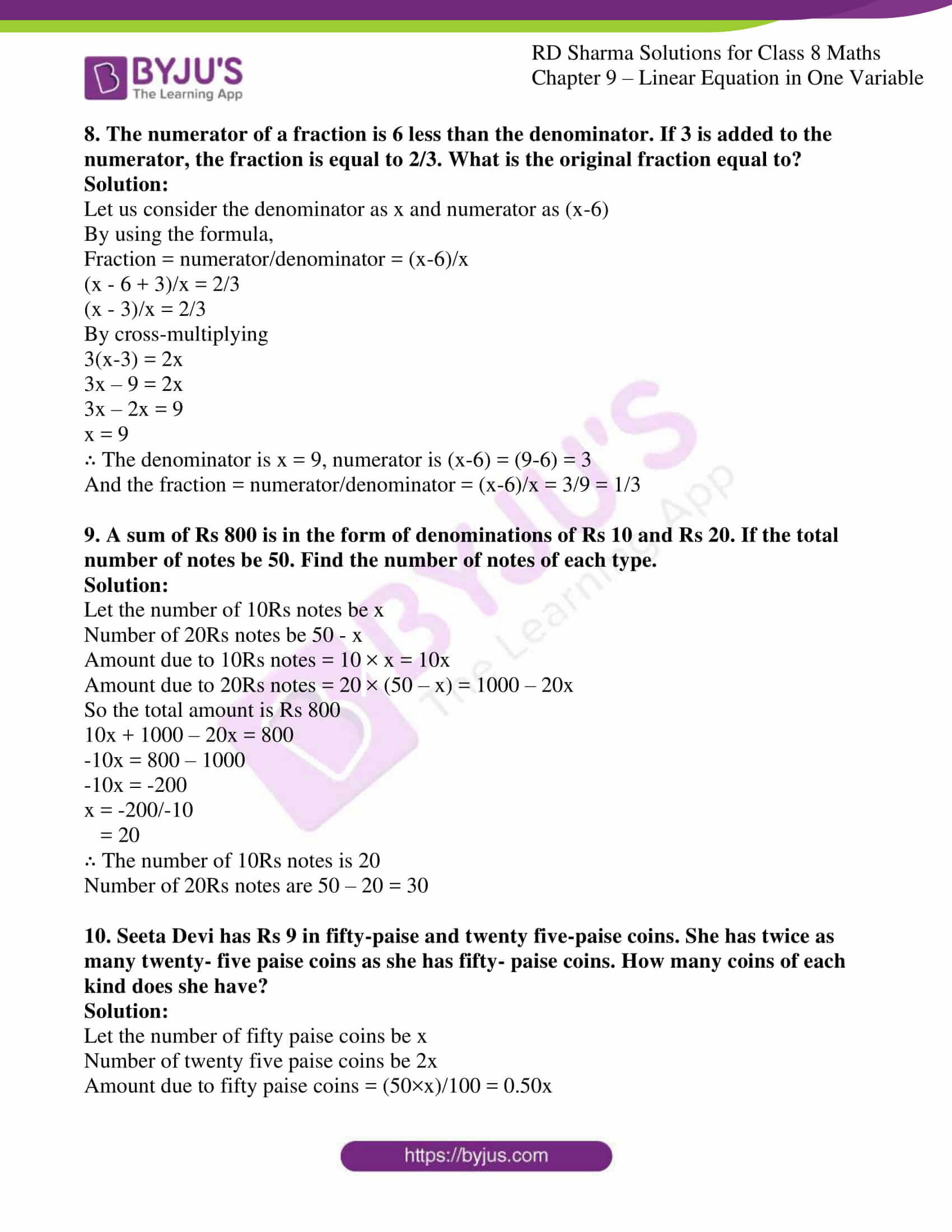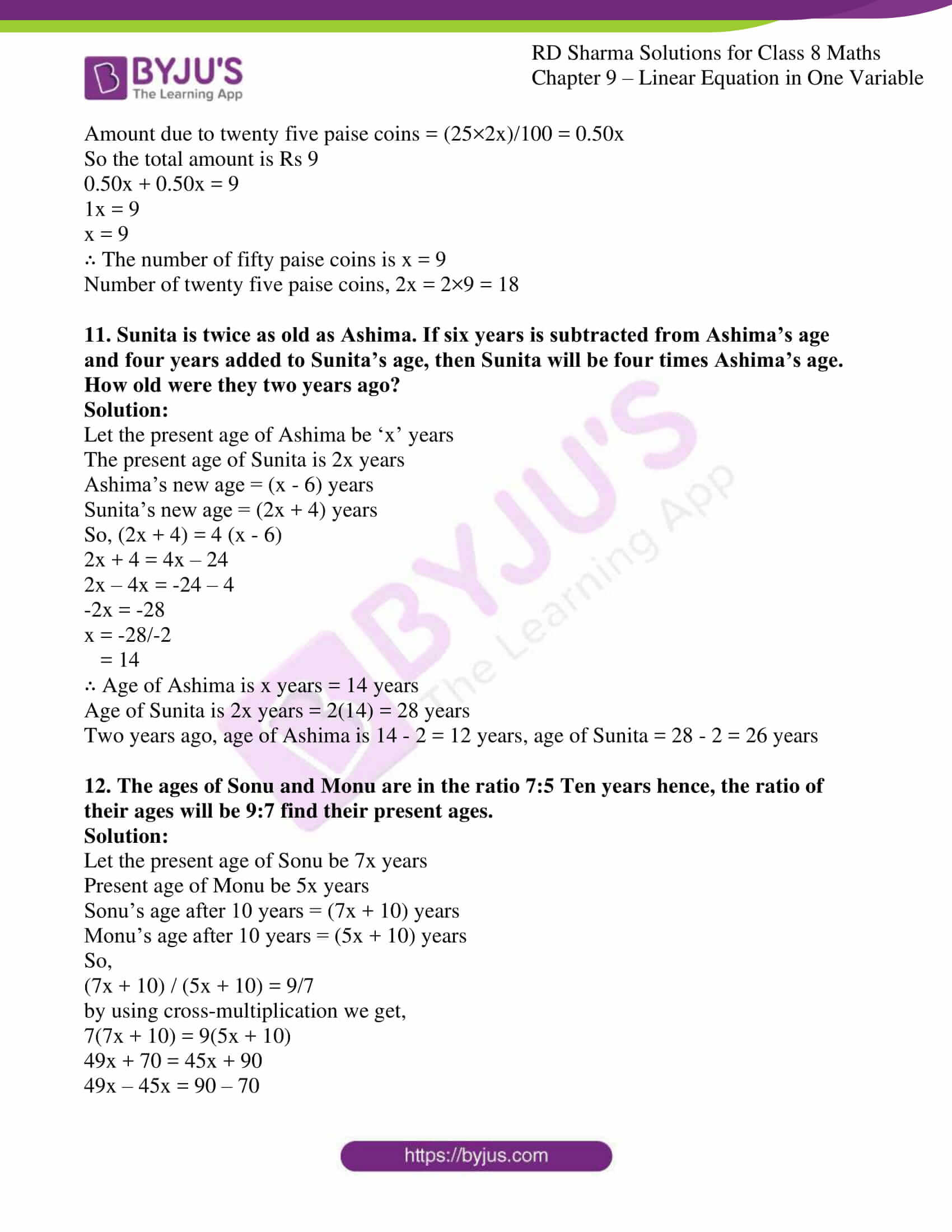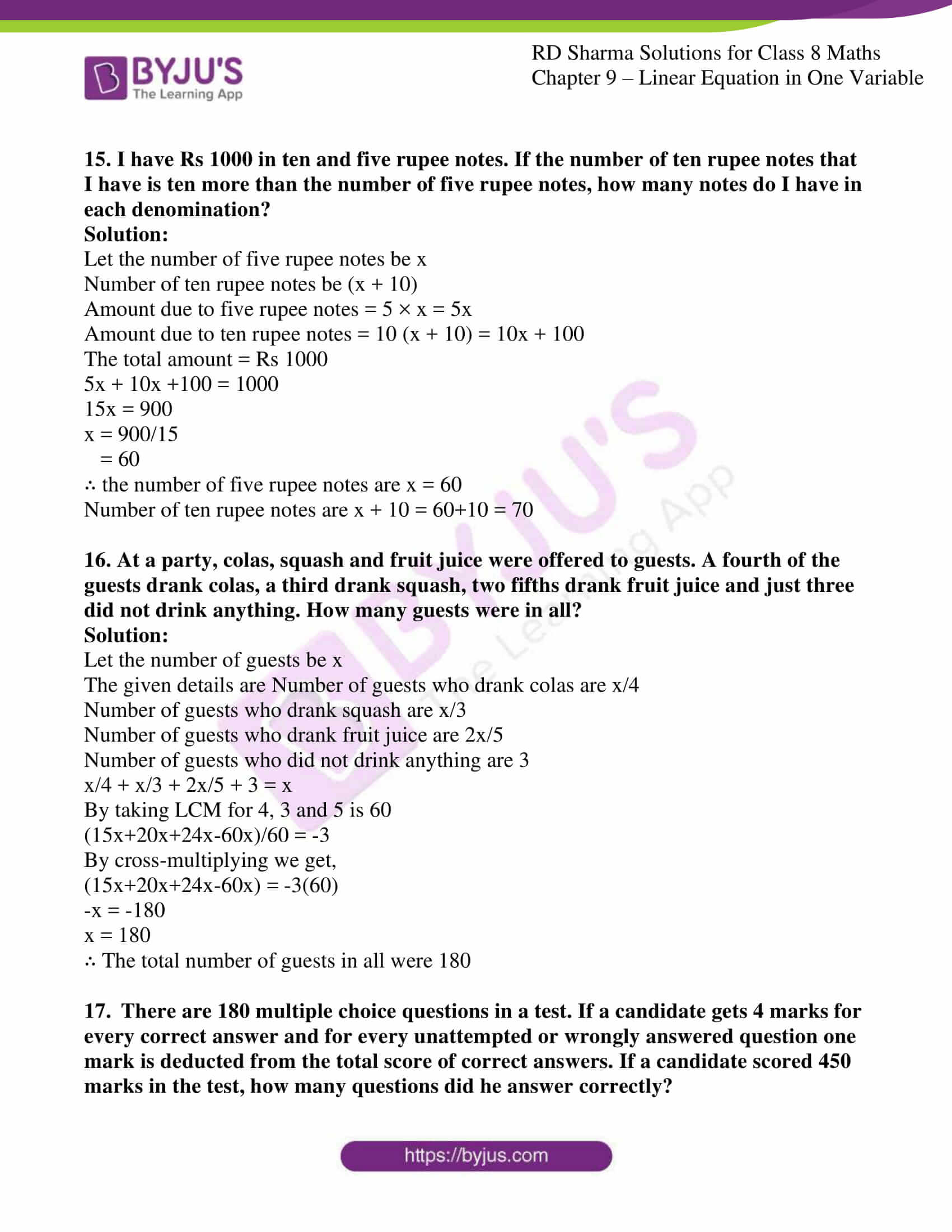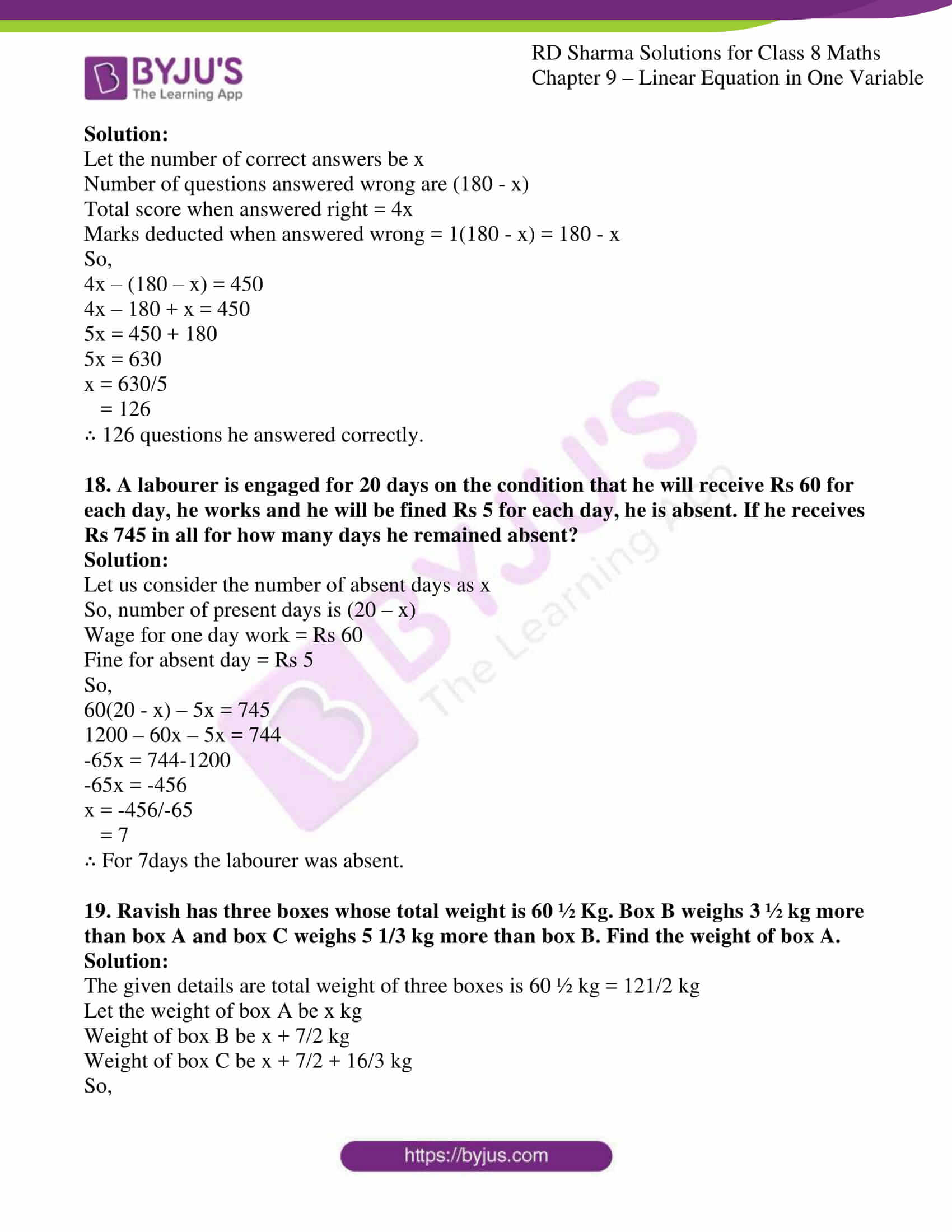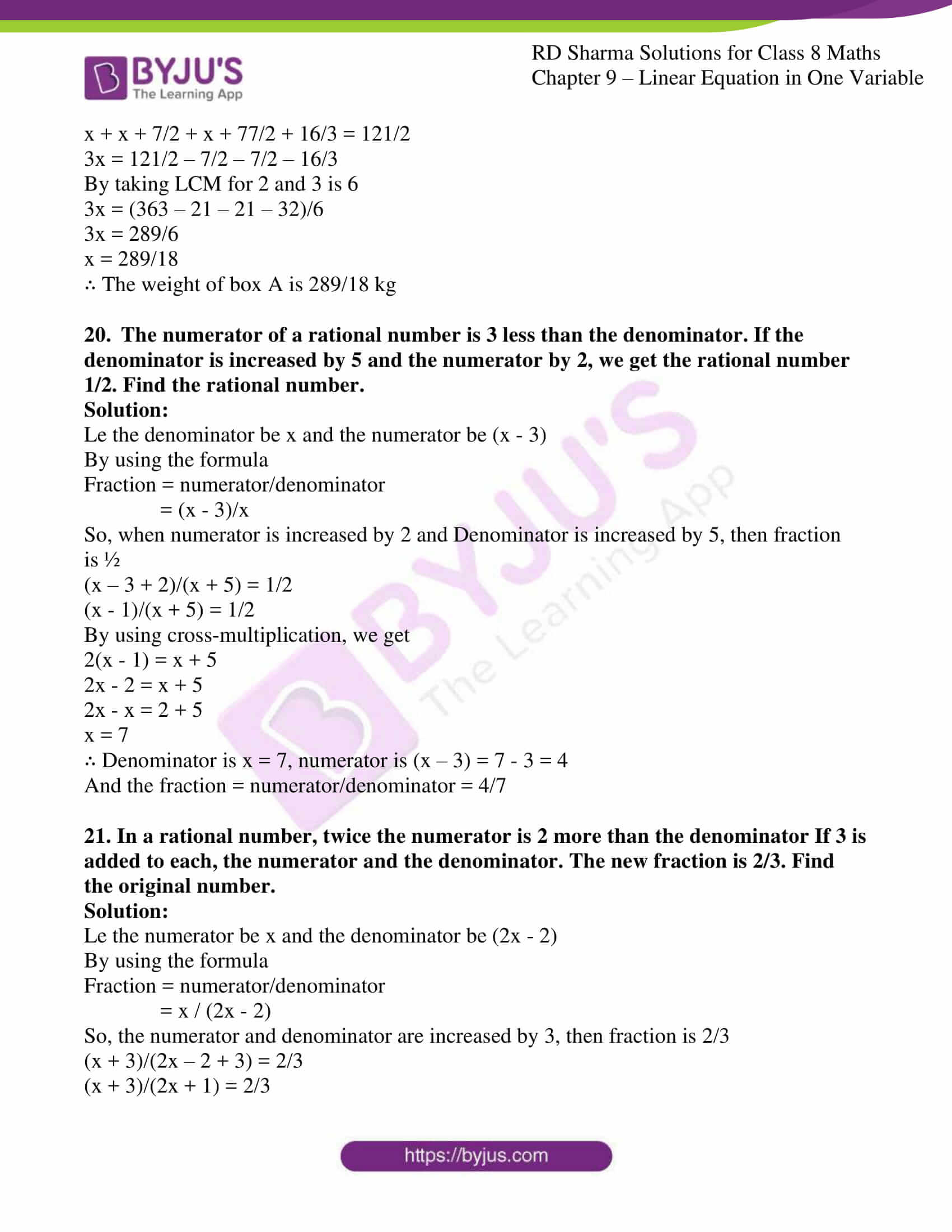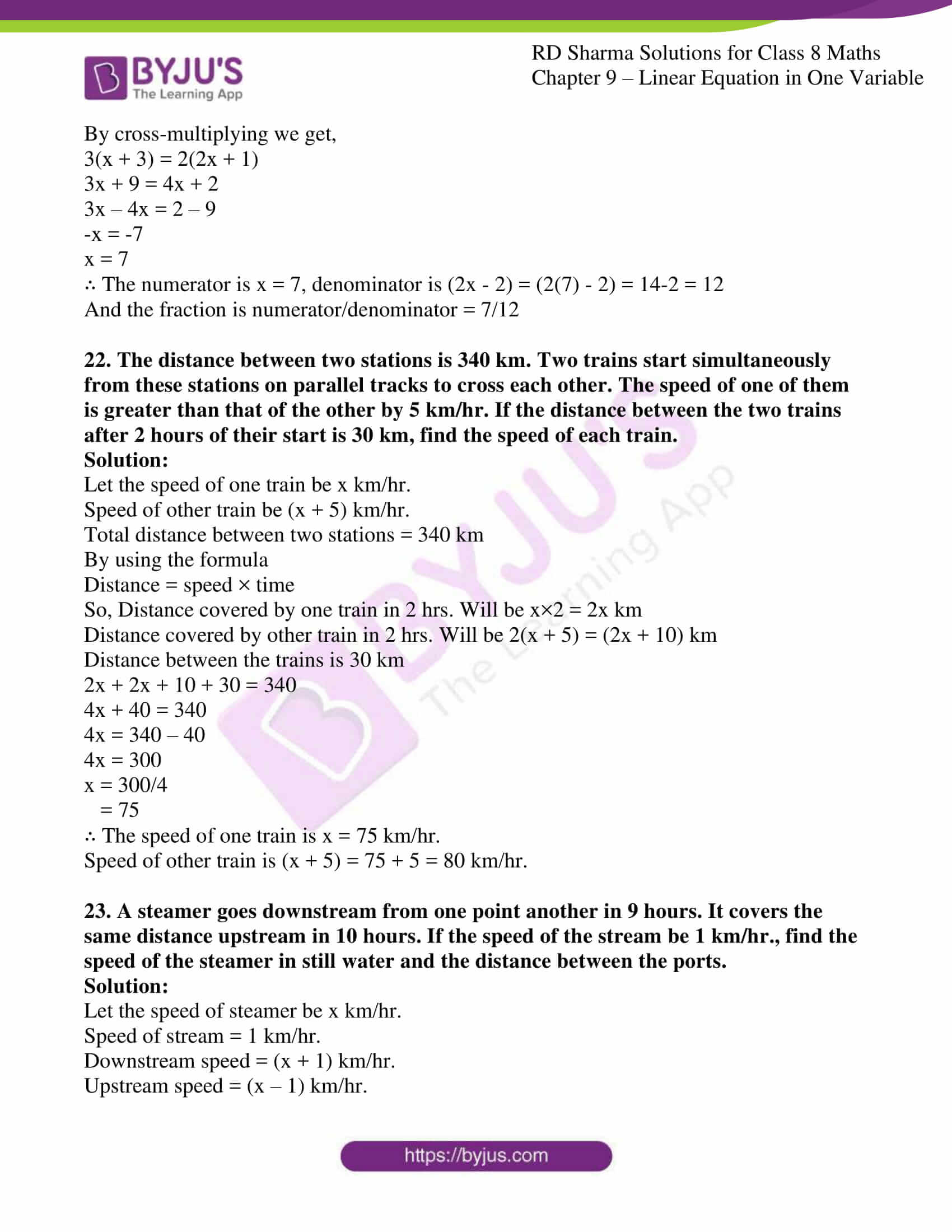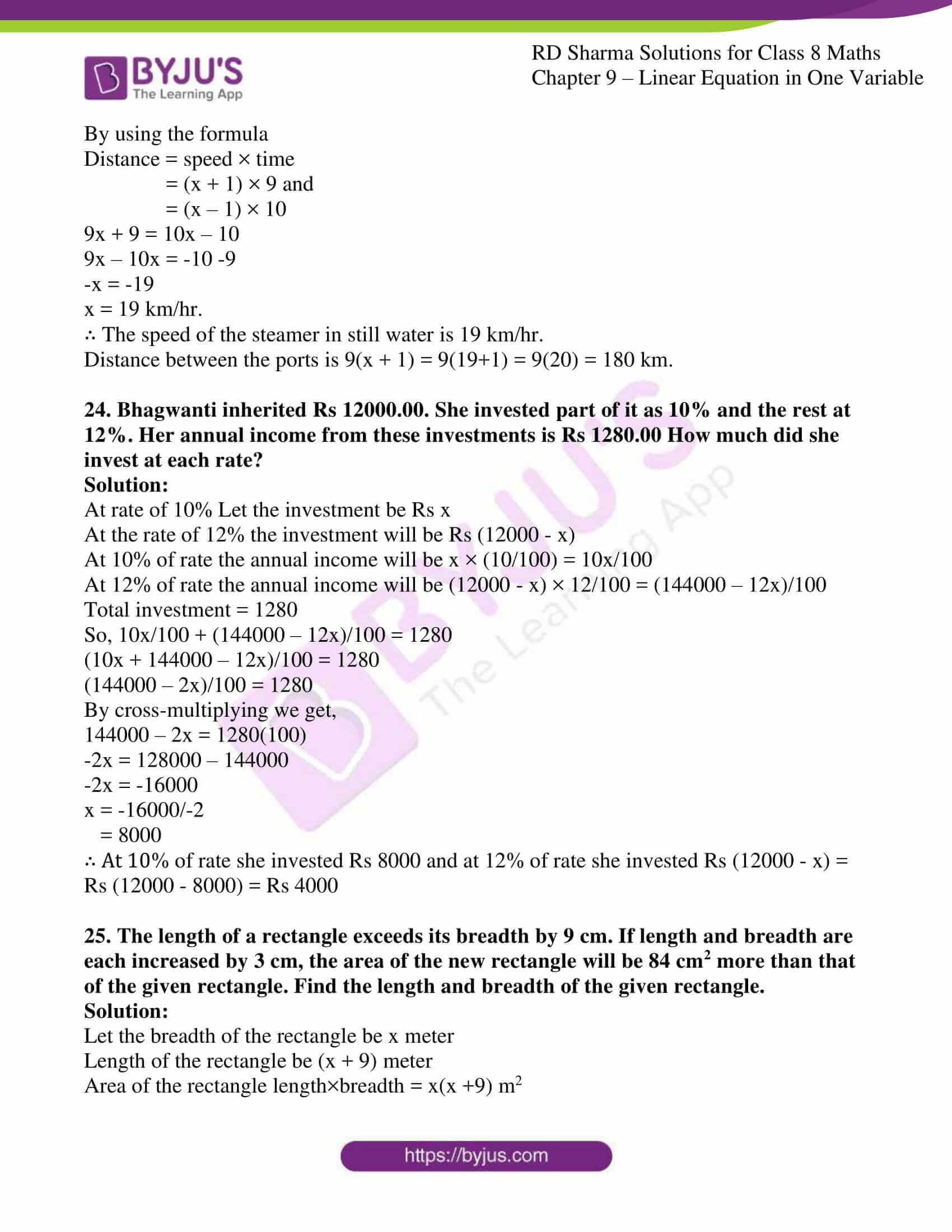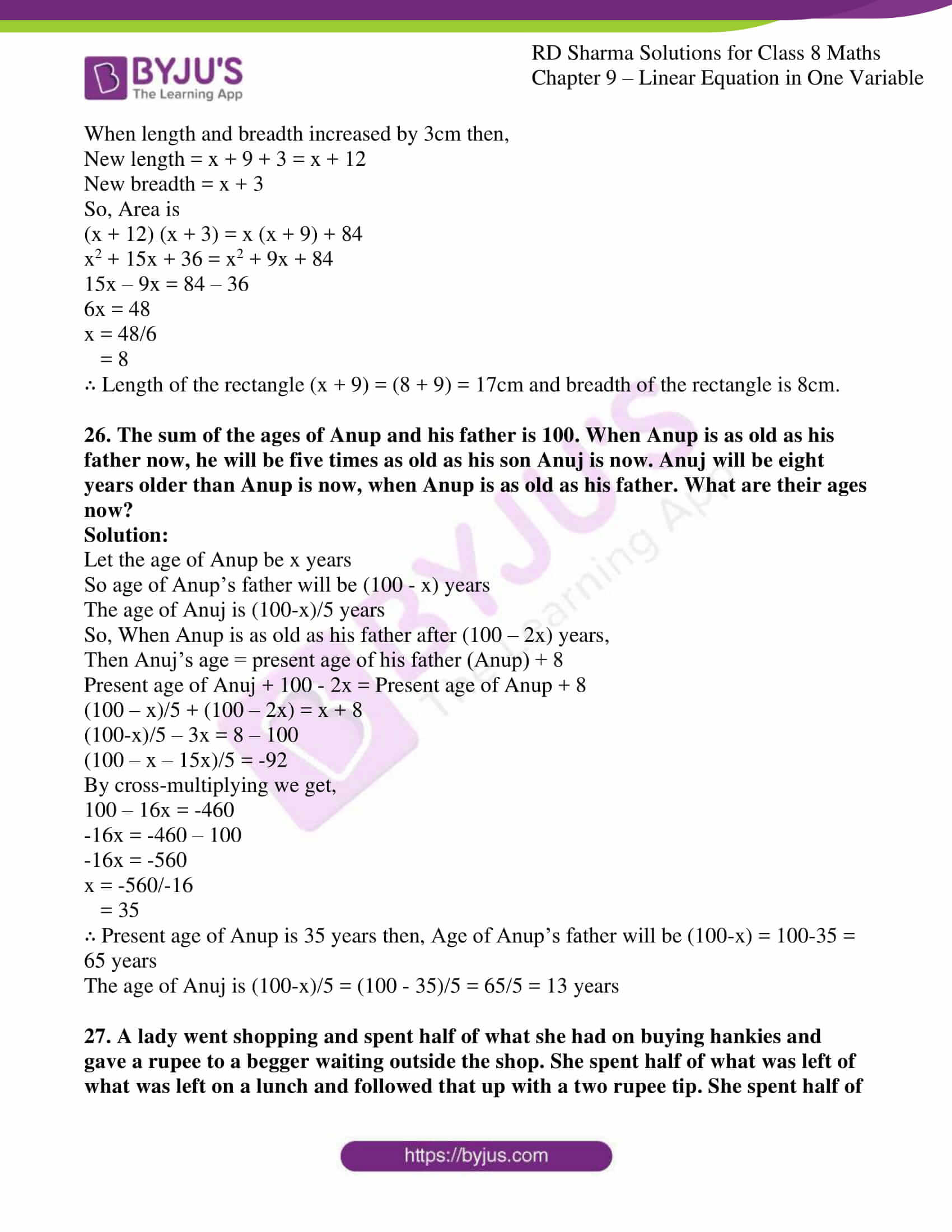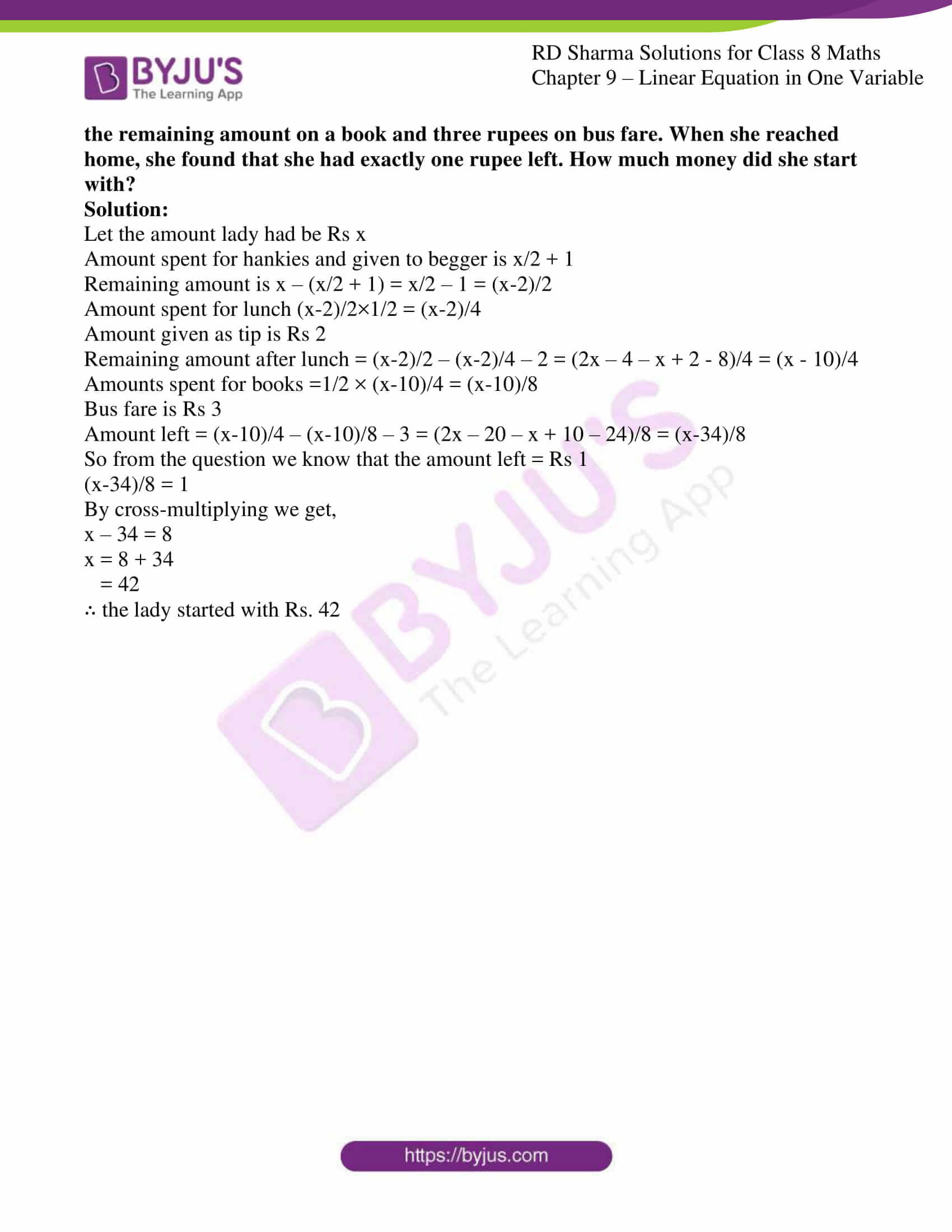### Access Answers to RD Sharma Solutions for Class 8 Maths Exercise 9.4 Chapter 9 Linear Equation in One Variable

1. Four-fifth of a number is more than three-fourth of the number by 4. Find the number.

Solution:

Let us consider the number as ‘x’

So, Three-fourth of the number is 3x/4

Fourth-fifth of the number is 4x/5

4x/5 – 3x/4 = 4

By taking LCM of 5 and 4 is 20

(16x – 15x)/20 = 4

By cross-multiplying we get,

16x – 15x = 4(20)

x = 80

∴ The number is 80.

2. The difference between the squares of two consecutive numbers is 31. Find the numbers.

Solution:

Let the two consecutive numbers be x and (x – 1)

So now,

x2 – (x-1)2 = 31

By using the formula (a-b)2 = a2 + b2 – 2ab

x2 – (x2 – 2x + 1) = 31

x2 – x2 + 2x – 1 = 31

2x – 1 = 31

2x = 31+1

2x = 32

x = 32/2

= 16

Two consecutive numbers are, x and (x-1) : 16 and (16-1) =15

∴ The two consecutive numbers are 16 and 15.

3. Find a number whose double is 45 greater than its half.

Solution:

Let us consider the number as ‘x’

So,

2x – x/2 = 45

(4x-x)/2 = 45

By cross-multiplying we get,

3x = 90

x = 90/3

= 30

∴ The number is 30.

4. Find a number such that when 5 is subtracted from 5 times that number, the result is 4 more than twice the number.

Solution:

Let us consider the number as ‘x’

Then, five times the number will be 5x

And, two times the number will be 2x

So,

5x – 5 = 2x + 4

5x – 2x = 5 + 4

3x = 9

x = 9/3

x = 3

∴ The number is 3.

5. A number whose fifth part increased by 5 is equal to its fourth part diminished by 5. Find the number.

Solution:

Let us consider the number as ‘x’

So,

x/5 + 5 = x/4 – 5

x/5 – x/4 = -5 – 5

By taking LCM for 5 and 4 which is 20

(4x-5x)/20 = -10

By cross-multiplying we get,

4x – 5x = -10(20)

-x = -200

x = 200

∴ The number is 200.

6. A number consists of two digits whose sum is 9. If 27 is subtracted from the number the digits are reversed. Find the number.

Solution:

We know that one of the digit be ‘x’

The other digit is 9-x

So, the two digit number is 10(9-x) + x

The number obtained after interchanging the digits is 10x + (9-x)

10(9-x) + x – 27 = 10x + (9-x)

Upon simplification,

90 – 10x + x – 27 = 10x + 9 – x

-10x + x – 10x + x = 9 – 90 + 27

-18x = -54

x = 54/18

= 9/3

= 3

The two digit number is 10(9-x) + x

Substituting the value of x we get,

10(9-x) + x

10(9 – 3) + 3

10(6) + 3

60+3

63

∴ The number is 63.

7. Divide 184 into two parts such that one-third of one part may exceed one-seventh of another part by 8.

Solution:

Let one of the number be ‘x’

The other number as 184 – x

So, One-third of one part may exceed one-seventh of another part by 8.

x/3 – (184-x)/7 = 8

LCM for 3 and 7 is 21

(7x – 552 + 3x)/21 = 8

By cross-multiplying we get,

(7x – 552 + 3x) = 8(21)

10x – 552 = 168

10x = 168 + 552

10x = 720

x = 720/10

= 72

∴ One of the number is 72 and other number is 184 – x => 184 – 72 = 112.

8. The numerator of a fraction is 6 less than the denominator. If 3 is added to the numerator, the fraction is equal to 2/3. What is the original fraction equal to?

Solution:

Let us consider the denominator as x and numerator as (x-6)

By using the formula,

Fraction = numerator/denominator = (x-6)/x

(x – 6 + 3)/x = 2/3

(x – 3)/x = 2/3

By cross-multiplying

3(x-3) = 2x

3x – 9 = 2x

3x – 2x = 9

x = 9

∴ The denominator is x = 9, numerator is (x-6) = (9-6) = 3

And the fraction = numerator/denominator = (x-6)/x = 3/9 = 1/3

9. A sum of Rs 800 is in the form of denominations of Rs 10 and Rs 20. If the total number of notes be 50. Find the number of notes of each type.

Solution:

Let the number of 10Rs notes be x

Number of 20Rs notes be 50 – x

Amount due to 10Rs notes = 10 × x = 10x

Amount due to 20Rs notes = 20 × (50 – x) = 1000 – 20x

So the total amount is Rs 800

10x + 1000 – 20x = 800

-10x = 800 – 1000

-10x = -200

x = -200/-10

= 20

∴ The number of 10Rs notes is 20

Number of 20Rs notes are 50 – 20 = 30

10. Seeta Devi has Rs 9 in fifty-paise and twenty five-paise coins. She has twice as many twenty- five paise coins as she has fifty- paise coins. How many coins of each kind does she have?

Solution:

Let the number of fifty paise coins be x

Number of twenty five paise coins be 2x

Amount due to fifty paise coins = (50×x)/100 = 0.50x

Amount due to twenty five paise coins = (25×2x)/100 = 0.50x

So the total amount is Rs 9

0.50x + 0.50x = 9

1x = 9

x = 9

∴ The number of fifty paise coins is x = 9

Number of twenty five paise coins, 2x = 2×9 = 18

11. Sunita is twice as old as Ashima. If six years is subtracted from Ashima’s age and four years added to Sunita’s age, then Sunita will be four times Ashima’s age. How old were they two years ago?

Solution:

Let the present age of Ashima be ‘x’ years

The present age of Sunita is 2x years

Ashima’s new age = (x – 6) years

Sunita’s new age = (2x + 4) years

So, (2x + 4) = 4 (x – 6)

2x + 4 = 4x – 24

2x – 4x = -24 – 4

-2x = -28

x = -28/-2

= 14

∴ Age of Ashima is x years = 14 years

Age of Sunita is 2x years = 2(14) = 28 years

Two years ago, age of Ashima is 14 – 2 = 12 years, age of Sunita = 28 – 2 = 26 years

12. The ages of Sonu and Monu are in the ratio 7:5 Ten years hence, the ratio of their ages will be 9:7 find their present ages.

Solution:

Let the present age of Sonu be 7x years

Present age of Monu be 5x years

Sonu’s age after 10 years = (7x + 10) years

Monu’s age after 10 years = (5x + 10) years

So,

(7x + 10) / (5x + 10) = 9/7

by using cross-multiplication we get,

7(7x + 10) = 9(5x + 10)

49x + 70 = 45x + 90

49x – 45x = 90 – 70

4x = 20

x = 20/4

= 5

∴ Present age of Sonu is 7x = 7(5) = 35years

Present age of Monu is 5x = 5(5) = 25years

13. Five years ago a man was seven times as old as his son. Five years hence, the father will be three times as old as his son. Find their present ages.

Solution:

Let the age of son five years ago be x years

The age of man five years ago be 7x years

After five years, son’s age is x + 5 years

After five years father’s age is 7x + 5 years

So, since five years, the relation in their ages are

7x + 5 + 5 = 3(x + 5 + 5)

7x + 10 = 3x + 15 + 15

7x + 10 = 3x + 30

7x – 3x = 30 – 10

4x = 20

x = 5

∴ Present father’s age is 7x + 5 = 7(5) + 5 = 35 + 5 = 40years

Present son’s age is x + 5 = 5 + 5 = 10years

14. I am currently 5 times as old as my son. In 6 years time I will be three times as old as he will be then. What are our ages now?

Solution:

Let the present son’s age be x years

Present father’s age be 5x years

Son’s age after 6 years = (x + 6) years

Fathers’ age after 6 years = (5x + 6) years

So,

5x + 6 = 3(x + 6)

5x + 6 = 3x + 18

5x – 3x = 18 – 6

2x = 12

x = 12/2

= 6

∴ present son’s age is x = 6years

Present father’s age is 5x = 5(6) = 30years

15. I have Rs 1000 in ten and five rupee notes. If the number of ten rupee notes that I have is ten more than the number of five rupee notes, how many notes do I have in each denomination?

Solution:

Let the number of five rupee notes be x

Number of ten rupee notes be (x + 10)

Amount due to five rupee notes = 5 × x = 5x

Amount due to ten rupee notes = 10 (x + 10) = 10x + 100

The total amount = Rs 1000

5x + 10x +100 = 1000

15x = 900

x = 900/15

= 60

∴ the number of five rupee notes are x = 60

Number of ten rupee notes are x + 10 = 60+10 = 70

16. At a party, colas, squash and fruit juice were offered to guests. A fourth of the guests drank colas, a third drank squash, two fifths drank fruit juice and just three did not drink anything. How many guests were in all?

Solution:

Let the number of guests be x

The given details are Number of guests who drank colas are x/4

Number of guests who drank squash are x/3

Number of guests who drank fruit juice are 2x/5

Number of guests who did not drink anything are 3

x/4 + x/3 + 2x/5 + 3 = x

By taking LCM for 4, 3 and 5 is 60

(15x+20x+24x-60x)/60 = -3

By cross-multiplying we get,

(15x+20x+24x-60x) = -3(60)

-x = -180

x = 180

∴ The total number of guests in all were 180

17. There are 180 multiple choice questions in a test. If a candidate gets 4 marks for every correct answer and for every unattempted or wrongly answered question one mark is deducted from the total score of correct answers. If a candidate scored 450 marks in the test, how many questions did he answer correctly?

Solution:

Let the number of correct answers be x

Number of questions answered wrong are (180 – x)

Total score when answered right = 4x

Marks deducted when answered wrong = 1(180 – x) = 180 – x

So,

4x – (180 – x) = 450

4x – 180 + x = 450

5x = 450 + 180

5x = 630

x = 630/5

= 126

∴ 126 questions he answered correctly.

18. A labourer is engaged for 20 days on the condition that he will receive Rs 60 for each day, he works and he will be fined Rs 5 for each day, he is absent. If he receives Rs 745 in all for how many days he remained absent?

Solution:

Let us consider the number of absent days as x

So, number of present days is (20 – x)

Wage for one day work = Rs 60

Fine for absent day = Rs 5

So,

60(20 – x) – 5x = 745

1200 – 60x – 5x = 744

-65x = 744-1200

-65x = -456

x = -456/-65

= 7

∴ For 7days the labourer was absent.

19. Ravish has three boxes whose total weight is 60 ½ Kg. Box B weighs 3 ½ kg more than box A and box C weighs 5 1/3 kg more than box B. Find the weight of box A.

Solution:

The given details are total weight of three boxes is 60 ½ kg = 121/2 kg

Let the weight of box A be x kg

Weight of box B be x + 7/2 kg

Weight of box C be x + 7/2 + 16/3 kg

So,

x + x + 7/2 + x + 77/2 + 16/3 = 121/2

3x = 121/2 – 7/2 – 7/2 – 16/3

By taking LCM for 2 and 3 is 6

3x = (363 – 21 – 21 – 32)/6

3x = 289/6

x = 289/18

∴ The weight of box A is 289/18 kg

20. The numerator of a rational number is 3 less than the denominator. If the denominator is increased by 5 and the numerator by 2, we get the rational number 1/2. Find the rational number.

Solution:

Le the denominator be x and the numerator be (x – 3)

By using the formula

Fraction = numerator/denominator

= (x – 3)/x

So, when numerator is increased by 2 and Denominator is increased by 5, then fraction is ½

(x – 3 + 2)/(x + 5) = 1/2

(x – 1)/(x + 5) = 1/2

By using cross-multiplication, we get

2(x – 1) = x + 5

2x – 2 = x + 5

2x – x = 2 + 5

x = 7

∴ Denominator is x = 7, numerator is (x – 3) = 7 – 3 = 4

And the fraction = numerator/denominator = 4/7

21. In a rational number, twice the numerator is 2 more than the denominator If 3 is added to each, the numerator and the denominator. The new fraction is 2/3. Find the original number.

Solution:

Le the numerator be x and the denominator be (2x – 2)

By using the formula

Fraction = numerator/denominator

= x / (2x – 2)

So, the numerator and denominator are increased by 3, then fraction is 2/3

(x + 3)/(2x – 2 + 3) = 2/3

(x + 3)/(2x + 1) = 2/3

By cross-multiplying we get,

3(x + 3) = 2(2x + 1)

3x + 9 = 4x + 2

3x – 4x = 2 – 9

-x = -7

x = 7

∴ The numerator is x = 7, denominator is (2x – 2) = (2(7) – 2) = 14-2 = 12

And the fraction is numerator/denominator = 7/12

22. The distance between two stations is 340 km. Two trains start simultaneously from these stations on parallel tracks to cross each other. The speed of one of them is greater than that of the other by 5 km/hr. If the distance between the two trains after 2 hours of their start is 30 km, find the speed of each train.

Solution:

Let the speed of one train be x km/hr.

Speed of other train be (x + 5) km/hr.

Total distance between two stations = 340 km

By using the formula

Distance = speed × time

So, Distance covered by one train in 2 hrs. Will be x×2 = 2x km

Distance covered by other train in 2 hrs. Will be 2(x + 5) = (2x + 10) km

Distance between the trains is 30 km

2x + 2x + 10 + 30 = 340

4x + 40 = 340

4x = 340 – 40

4x = 300

x = 300/4

= 75

∴ The speed of one train is x = 75 km/hr.

Speed of other train is (x + 5) = 75 + 5 = 80 km/hr.

23. A steamer goes downstream from one point another in 9 hours. It covers the same distance upstream in 10 hours. If the speed of the stream be 1 km/hr., find the speed of the steamer in still water and the distance between the ports.

Solution:

Let the speed of steamer be x km/hr.

Speed of stream = 1 km/hr.

Downstream speed = (x + 1) km/hr.

Upstream speed = (x – 1) km/hr.

By using the formula

Distance = speed × time

= (x + 1) × 9 and

= (x – 1) × 10

9x + 9 = 10x – 10

9x – 10x = -10 -9

-x = -19

x = 19 km/hr.

∴ The speed of the steamer in still water is 19 km/hr.

Distance between the ports is 9(x + 1) = 9(19+1) = 9(20) = 180 km.

24. Bhagwanti inherited Rs 12000.00. She invested part of it as 10% and the rest at 12%. Her annual income from these investments is Rs 1280.00 How much did she invest at each rate?

Solution:

At rate of 10% Let the investment be Rs x

At the rate of 12% the investment will be Rs (12000 – x)

At 10% of rate the annual income will be x × (10/100) = 10x/100

At 12% of rate the annual income will be (12000 – x) × 12/100 = (144000 – 12x)/100

Total investment = 1280

So, 10x/100 + (144000 – 12x)/100 = 1280

(10x + 144000 – 12x)/100 = 1280

(144000 – 2x)/100 = 1280

By cross-multiplying we get,

144000 – 2x = 1280(100)

-2x = 128000 – 144000

-2x = -16000

x = -16000/-2

= 8000

∴ At 10% of rate she invested Rs 8000 and at 12% of rate she invested Rs (12000 – x) = Rs (12000 – 8000) = Rs 4000

25. The length of a rectangle exceeds its breadth by 9 cm. If length and breadth are each increased by 3 cm, the area of the new rectangle will be 84 cm2 more than that of the given rectangle. Find the length and breadth of the given rectangle.

Solution:

Let the breadth of the rectangle be x meter

Length of the rectangle be (x + 9) meter

Area of the rectangle length×breadth = x(x +9) m2

When length and breadth increased by 3cm then,

New length = x + 9 + 3 = x + 12

New breadth = x + 3

So, Area is

(x + 12) (x + 3) = x (x + 9) + 84

x2 + 15x + 36 = x2 + 9x + 84

15x – 9x = 84 – 36

6x = 48

x = 48/6

= 8

∴ Length of the rectangle (x + 9) = (8 + 9) = 17cm and breadth of the rectangle is 8cm.

26. The sum of the ages of Anup and his father is 100. When Anup is as old as his father now, he will be five times as old as his son Anuj is now. Anuj will be eight years older than Anup is now, when Anup is as old as his father. What are their ages now?

Solution:

Let the age of Anup be x years

So age of Anup’s father will be (100 – x) years

The age of Anuj is (100-x)/5 years

So, When Anup is as old as his father after (100 – 2x) years,

Then Anuj’s age = present age of his father (Anup) + 8

Present age of Anuj + 100 – 2x = Present age of Anup + 8

(100 – x)/5 + (100 – 2x) = x + 8

(100-x)/5 – 3x = 8 – 100

(100 – x – 15x)/5 = -92

By cross-multiplying we get,

100 – 16x = -460

-16x = -460 – 100

-16x = -560

x = -560/-16

= 35

∴ Present age of Anup is 35 years then, Age of Anup’s father will be (100-x) = 100-35 = 65 years

The age of Anuj is (100-x)/5 = (100 – 35)/5 = 65/5 = 13 years

27. A lady went shopping and spent half of what she had on buying hankies and gave a rupee to a begger waiting outside the shop. She spent half of what was left of what was left on a lunch and followed that up with a two rupee tip. She spent half of the remaining amount on a book and three rupees on bus fare. When she reached home, she found that she had exactly one rupee left. How much money did she start with?

Solution:

Amount spent for hankies and given to begger is x/2 + 1

Remaining amount is x – (x/2 + 1) = x/2 – 1 = (x-2)/2

Amount spent for lunch (x-2)/2×1/2 = (x-2)/4

Amount given as tip is Rs 2

Remaining amount after lunch = (x-2)/2 – (x-2)/4 – 2 = (2x – 4 – x + 2 – 8)/4 = (x – 10)/4

Amounts spent for books =1/2 × (x-10)/4 = (x-10)/8

Bus fare is Rs 3

Amount left = (x-10)/4 – (x-10)/8 – 3 = (2x – 20 – x + 10 – 24)/8 = (x-34)/8

So from the question we know that the amount left = Rs 1

(x-34)/8 = 1

By cross-multiplying we get,

x – 34 = 8

x = 8 + 34

= 42

∴ the lady started with Rs. 42

#### 1 Comment

1. K.V.Vishruthi

The byjus is absolutely great!! But you ,byjus staffs can make the error connection a bit faster and can solve the sums little more easier and I. A better way so students like us can understand much more easier.